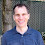## Thursday, February 24, 2022

### I will be on instagram/If you have two reals in a box- Answer (Guest Post by David Marcus)

I will be on instragram:

We,  Prof. Mohammad Hajiaghayi and Prof. William Gasarch plan to have an Instagram Live at @mhajiaghayi this SAT FEB 26, 1:30PM EDT (in English).

We talk about our lives as well as blogging, research, and how to inspire young minds. Please join if you can.

#PvsNP #blogging #youngminds #instagram

-------------------------------------

This is the answer to the problem posted in the last post which was a guest post by David Marcus. This post is also a guest post by him.

We restate the problem:

-------------------------------------------------

PICK THE LARGEST NUMBER:

There are two distinct reals in a box (how do they fit! aren't reals infinite!). They are called the first and second number.

You want to pick the larger one. You can pick one of the numbers at random and look at it

before deciding which one you want.

Is there a strategy that will win with probability > 1/2.

CLARIFICATIONS:

1) We do not want a strategy that does well on average or in the long run. Even if the games is played just once, we want the probability of winning to be > 1/2.

2) It must have prob of winning > 1/2 for EVERY pair of reals.

3) Here is an example of a strategy that does NOT work: Pick the first number with prob 1/2. If its positive then keep it, else dump it. If the numbers are{1,2} this only works half the time.

-------------------------------------------------

There is a strategy. In fact, we give two.

STRATEGY ONE

Before looking at any number, pick a number x on your own from the reals according to a distribution with full support (every open interval has prob greater than 0). Then pick a number from the box (prob 1/2-1/2), which we call y. If x<y then keep y. If y\le x then take the other number.

If x is between the two numbers, then the strategy works. If not then the strategy does not hurt, so the prob in that case is 1/2. Hence you do better than 1/2.

STRATEGY TWO

Let f: R--> (0,1) be strictly increasing. Pick a number from the box, call it y. Keep it with prob f(y).

1.These were the ones given in the two comments of the original post.

Also, those are not two different strategies but the same one said in two different ways. The f in the second strategy is the cdf of the distribution from the first strategy.

1.I'd rather say the two strategies are "related" rather than being the "same". I noted that in my draft, but Bill edited it out!

2.What about picking one of the two with probability 1/2, and if its fractional part is >= 0.5 (<= 0.5 for negative numbers) then keep it, otherwise pick the other?
(I'm totally unfamiliar with probabilities)

1.Knowing that strategy, always having the numbers be 1 and 2 gives you a 50/50 chance.

3.Consider, e.g., 1.7 and 2.0, then you will always choose 1.7 so your probability of winning is zero. I guess that the point of the problem was that any deterministic strategy is not better than 1/2 in the worst case while an easy probabilistic one may be better than 1/2 uniformly.

4.Evangelos Georgiadis (EG)8:48 PM, March 02, 2022

Intriguing. A few clarification questions on this.
(a) how would you go about proving this? too obvious?
(b) what is the provenance of this problem?
A previous commentator stated that the late Tom Cover covered this (and perhaps a more generalized) problem in a conference? source/proceedings?

1.Are you asking how to prove that the solutions work?

Strategy one: Let p be the probability that x is between the two numbers. So, p > 0. If x is between the two numbers, then you end up with the larger number. Otherwise, you have probability 1/2 of ending up with the larger number. So, your overall probability of ending up with the larger number is p + ( 1 - p ) ( 1/2 ) = 1/2 + p ( 1 - 1/2 ) = 1/2 + p/2 > 1/2.

Strategy two: Let a be the smaller number and b be the larger number. If you select a from the box, you have probability 1 - f(a) of ending up with the larger number. If you select b from the box, you have probability f(b) of ending up with the larger number. So, your overall probability of ending up with the larger number is ( 1 - f(a) ) / 2 + f(b) / 2 = 1/2 + ( f(b) - f(a) ) / 2 > 1/2.

I don't know the original source of the puzzle, nor does the person that I learned the puzzle from.

5.Evangelos Georgiadis (EG)9:59 PM, March 02, 2022

regarding (b).
https://isl.stanford.edu/people/cover/papers/paper73.pdf
Seems like a pointer. The link to the optimal stopping problem
is mentioned. @Marcus did you look into this?

1.I had not seen that. He doesn't say that he originated the problem.

2.I think the problem is older than that paper.